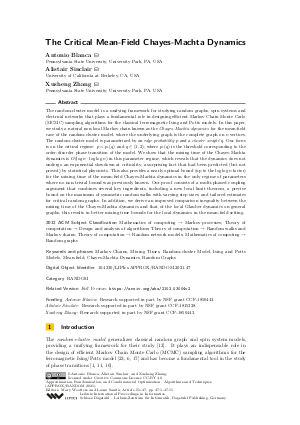Document# The Critical Mean-Field Chayes-Machta Dynamics

### Authors Antonio Blanca, Alistair Sinclair, Xusheng Zhang## File

LIPIcs.APPROX-RANDOM.2021.47.pdf
• Filesize: 0.65 MB
• 15 pages

## Cite As

Antonio Blanca, Alistair Sinclair, and Xusheng Zhang. The Critical Mean-Field Chayes-Machta Dynamics. In Approximation, Randomization, and Combinatorial Optimization. Algorithms and Techniques (APPROX/RANDOM 2021). Leibniz International Proceedings in Informatics (LIPIcs), Volume 207, pp. 47:1-47:15, Schloss Dagstuhl - Leibniz-Zentrum für Informatik (2021)
https://doi.org/10.4230/LIPIcs.APPROX/RANDOM.2021.47

## Abstract

The random-cluster model is a unifying framework for studying random graphs, spin systems and electrical networks that plays a fundamental role in designing efficient Markov Chain Monte Carlo (MCMC) sampling algorithms for the classical ferromagnetic Ising and Potts models. In this paper, we study a natural non-local Markov chain known as the Chayes-Machta dynamics for the mean-field case of the random-cluster model, where the underlying graph is the complete graph on n vertices. The random-cluster model is parametrized by an edge probability p and a cluster weight q. Our focus is on the critical regime: p = p_c(q) and q ∈ (1,2), where p_c(q) is the threshold corresponding to the order-disorder phase transition of the model. We show that the mixing time of the Chayes-Machta dynamics is O(log n ⋅ log log n) in this parameter regime, which reveals that the dynamics does not undergo an exponential slowdown at criticality, a surprising fact that had been predicted (but not proved) by statistical physicists. This also provides a nearly optimal bound (up to the log log n factor) for the mixing time of the mean-field Chayes-Machta dynamics in the only regime of parameters where no non-trivial bound was previously known. Our proof consists of a multi-phased coupling argument that combines several key ingredients, including a new local limit theorem, a precise bound on the maximum of symmetric random walks with varying step sizes, and tailored estimates for critical random graphs. In addition, we derive an improved comparison inequality between the mixing time of the Chayes-Machta dynamics and that of the local Glauber dynamics on general graphs; this results in better mixing time bounds for the local dynamics in the mean-field setting.

## Subject Classification

##### ACM Subject Classification
• Mathematics of computing → Markov processes
• Theory of computation → Design and analysis of algorithms
• Theory of computation → Random walks and Markov chains
• Theory of computation → Random network models
• Mathematics of computing → Random graphs
##### Keywords
• Markov Chains
• Mixing Times
• Random-cluster Model
• Ising and Potts Models
• Mean-field
• Chayes-Machta Dynamics
• Random Graphs

## Metrics

• Access Statistics
• Total Accesses (updated on a weekly basis)
0

## References

1. V. Beffara and H. Duminil-Copin. The self-dual point of the two-dimensional random-cluster model is critical for q ≥ 1. Probability Theory and Related Fields, 153:511-542, 2012.2. A. Blanca. Random-cluster dynamics. PhD thesis, UC Berkeley, 2016.3. A. Blanca and R. Gheissari. Random-cluster dynamics on random regular graphs in tree uniqueness. Communications in Mathematical Physics, 2021.4. A. Blanca, R. Gheissari, and E. Vigoda. Random-cluster dynamics in ℤ²: rapid mixing with general boundary conditions. Annals of Applied Probability, 30(1):418-459, 2020.5. A. Blanca and A. Sinclair. Dynamics for the mean-field random-cluster model. Proceedings of the 19th International Workshop on Randomization and Computation (RANDOM), pages 528-543, 2015.6. A. Blanca and A. Sinclair. Random-Cluster Dynamics in ℤ². Probability Theory and Related Fields, 168:821-847, 2017.7. Antonio Blanca, Alistair Sinclair, and Xusheng Zhang. The critical mean-field chayes-machta dynamics, 2021. URL: http://arxiv.org/abs/2102.03004.
8. B. Bollobás, G.R. Grimmett, and S. Janson. The random-cluster model on the complete graph. Probability Theory and Related Fields, 104(3):283-317, 1996.9. L. Chayes and J. Machta. Graphical representations and cluster algorithms II. Physica A, 254:477-516, 1998.10. H. Duminil-Copin, M. Gagnebin, M. Harel, I. Manolescu, and V. Tassion. Discontinuity of the phase transition for the planar random-cluster and Potts models with q > 4. Annales de l'ENS, 2016. To Appear.11. H. Duminil-Copin, V. Sidoravicius, and V. Tassion. Continuity of the Phase Transition for Planar Random-Cluster and Potts Models with 1 ≤ q ≤ 4. Communications in Mathematical Physics, 349(1):47-107, 2017.12. C.M. Fortuin and P.W. Kasteleyn. On the random-cluster model I. Introduction and relation to other models. Physica, 57(4):536-564, 1972.13. A. Galanis, D. Štefankovič, and E. Vigoda. Swendsen-Wang algorithm on the mean-field Potts model. Proceedings of the 19th International Workshop on Randomization and Computation (RANDOM), pages 815-828, 2015.14. T. Garoni. Personal communication, 2015.15. R. Gheissari and E. Lubetzky. Quasi-polynomial mixing of critical two-dimensional random cluster models. Random Structures & Algorithms, 56(2):517-556, 2020.16. R. Gheissari, E. Lubetzky, and Y. Peres. Exponentially slow mixing in the mean-field Swendsen-Wang dynamics. In Proceedings of the 29th Annual ACM-SIAM Symposium on Discrete Algorithms (SODA), pages 1981-1988. SIAM, 2018.17. H. Guo and M. Jerrum. Random cluster dynamics for the Ising model is rapidly mixing. In Proceedings of the 28th Annual ACM-SIAM Symposium on Discrete Algorithms (SODA), pages 1818-1827. SIAM, 2017.18. D.A. Levin and Y. Peres. Markov Chains and Mixing Times. MBK. American Mathematical Society, 2017. URL: https://books.google.com/books?id=f208DwAAQBAJ.
19. Y. Long, A. Nachmias, W. Ning, and Y. Peres. A power law of order 1/4 for critical mean-field Swendsen-Wang dynamics. Memoirs of the American Mathematical Society, 232(1092), 2011.20. Malwina Luczak and Tomasz Luczak. The phase transition in the cluster-scaled model of a random graph. Random Structures & Algorithms, 28(2):215-246, 2006.21. A.B. Mukhin. Local limit theorems for lattice random variables. Theory of Probability & Its Applications, 36(4):698-713, 1992.22. R.H. Swendsen and J.S. Wang. Nonuniversal critical dynamics in Monte Carlo simulations. Physical Review Letters, 58:86-88, 1987.23. M. Ullrich. Swendsen-Wang is faster than single-bond dynamics. SIAM Journal on Discrete Mathematics, 28(1):37-48, 2014.X

Feedback for Dagstuhl Publishing